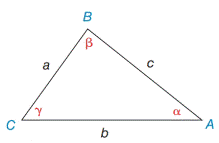Chapter 11.4, Problem 2E### Elementary Geometry for College St...

6th Edition
Daniel C. Alexander + 1 other
ISBN: 9781285195698

#### Solutions

Chapter
Section### Elementary Geometry for College St...

6th Edition
Daniel C. Alexander + 1 other
ISBN: 9781285195698
Textbook Problem
1 views

# In Exercises 1 and 2, use the given information to find an expression for the area of Δ A B C . Give the answer in a form such as A = 1 2 ( 3 ) ( 4 )  sin 32 ∘ . See the figure for Exercises 1- 8.a) b = 7.3 ,   c = 8.6 ,  and  α = 38 ∘ b) a = 5.3 ,   c = 8.4 ,   α = 36 ∘  and  γ = 87 ∘Exercises 1-8

To determine

a)

To find:

The area of the ABC in the form of A=12(a)(b) sin α

If a=7.3, b=8.6, and α=38

Explanation

1) Approach:

The area of an acute triangle equals one-half the product of the lengths of two sides and the sine of the included angle.

The area of the triangle ABC is given by,

A=12absinα

2) Calculation:

Given,

In triangle ABC,

a=7.3b=8.6 andα=38

The area of the triangle ABC A=12absinα

A=12(7

To determine

To find:

The area of the ABC in the form of A=12(a)(b) sin γ

If a=5.3, b=8.4, α=36 and γ=87

### Still sussing out bartleby?

Check out a sample textbook solution.

See a sample solution

#### The Solution to Your Study Problems

Bartleby provides explanations to thousands of textbook problems written by our experts, many with advanced degrees!

Get Started

#### Completely factor each of the following.

Mathematical Applications for the Management, Life, and Social Sciences

#### Equations Solve the equation graphically. 140. x+4=x25

Precalculus: Mathematics for Calculus (Standalone Book)

#### True or False: Partial fractions may be used for .

Study Guide for Stewart's Single Variable Calculus: Early Transcendentals, 8th